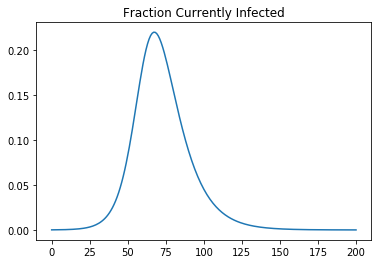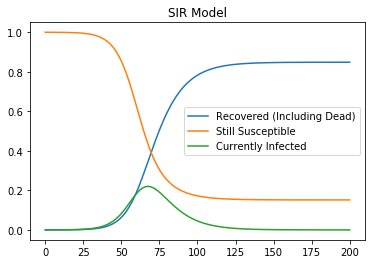# A Note: How Much Difference Does Heterogeneity Make in the Susceptible, Infected, Recovered (or Not) Model?¶

It turns out it makes a significant amount of difference:

## The Basic Model¶

### The Framework¶

Assume a continuum of people, indexed by j ∈ [0, 1].

• For each j there is a measure of how gregarious/vulnerable the people at that j are: how many others they instantaneously contact: $g_j$.

• For each j, there are measures of how many of the people at that j are currently, at each time t, susceptible, infected, and recovered: $s_{jt} + i_{jt} + r_{jt} = 1$.

• People recover from being infected at rate $\rho$ (including dying): $\frac{dr_{jt}}{dt} = \rho i_{jt}$

• A fraction $\delta$ of the recovered are dead: $d_{jt} = \delta r_{jt}$

• People get infected if they are susceptible and if one of the $g_j$ they contact is currently infected: $\frac{di_{jt}}{dt} = g_j s_{jt} I_t - \rho i_{jt}$

• The total number of currently infected: $I_t = \int_0^1 {i_{jt} dj}$

• The total number of currently recovered: $R_t = \int_0^1 {r_{jt} dj}$

• The total number of currently susceptiable: $S_t = \int_0^1 {s_{jt} dj}$

• The total number of currently dead is: $D_t = \delta R_t$

### Initial Conditions¶

• Initially, before the epidemic: $s_{j0} = S_0 = 1 - I_0$

• Initially, before the epidemic: $r_{j0} = R_0 = 0$

• Initially, before the epidemic: $i_{j0} = I_0$

### Parameters¶

There is one initial infection rate $I_0$, one recovery rate $\rho$, one share of the recovered who are dead $\delta$, and a continuum of gregarious/vulnerable rates $g_j$.

For simplicity let us cut our number of parameters to five and assign the $g_j$ linearly based on two end parameters $g_{min}$ and $g_{max}$: $g_j = j \left( g^{max} \right) + (1-j) \left( g^{min} \right)$

In :
import numpy as np
import scipy as sp
import pandas as pd
import matplotlib as mpl
import matplotlib.pyplot as plt
%matplotlib inline

# paramaters

time = 200                    # time periods we run the simulation for
bins = 101                    # number of bins into which the population is divided

R_zero_min = 1.0              # R_0 for least gregarious/infective
R_zero_max = 4.0              # R_0 for most gregarious/infective

rho = 0.1                     # recovery rate
g_min = R_zero_min * rho      # minimum gregariousness/infectiveness
g_max = R_zero_max * rho      # maximum gregariousness/infectiveness
delta = 0.01                  # death rate

I_zero = 0.0001               # initial infection rate

# averages over the population at the current moment of S, I, R—
# susceptible, infected, recovered (or dead), initialized to their
# time-zero values
I_tavg = I_zero
R_tavg = 0
S_tavg = 1 - I_zero

# time series for the entire population of the fractions S, I, R;
# initialized with their time-zero values
T_pop = 
R_pop = [R_tavg]
I_pop = [I_tavg]
S_pop = [S_tavg]

# heterogeneity across the population in gregariousness/infectiveness
G = np.linspace(g_min, g_max, 101)

# initial conditions across the population, for all j:
R_0 = 0*G
I_0 = 0*G + I_zero
S_0 = 1 - I_zero

# arrays to hold current-time population heterogeneity—all j at the current
# moment of time—initialized to their values at time zero:
R = R_0
I = I_0
S = S_0

# arrays to hold all the numbers—for all j, and for all t—for when we want
# to look at them later:
R_array = [R]
I_array = [I]
S_array = [S]

# loop to calculate all the numbers:
for t in range(0, time):
R = R + rho * I
I = (1-rho) * I + I_tavg * G * S
S = - (R + I - 1)

# subloop to calculate average S, I, R across all j at the current
# moment of time:
I_tavg = 0
R_tavg = 0
S_tavg = 0
for j in range(0, bins):
I_tavg = I_tavg + I[j]/bins
R_tavg = R_tavg + R[j]/bins
S_tavg = S_tavg + S[j]/bins

# update the full arrays with the current numbers for all j:
R_array = R_array + [R]
I_array = I_array + [I]
S_array = S_array + [S]

# update the time series of population averages:
T_pop = T_pop + [t+1]
R_pop = R_pop + [R_tavg]
S_pop = S_pop + [S_tavg]
I_pop = I_pop + [I_tavg]

pd.DataFrame(I_pop).plot(title = "Fraction Currently Infected", legend = False)

Out:
<matplotlib.axes._subplots.AxesSubplot at 0x120c2f2d0>In :
Results = [R_pop, S_pop,I_pop]
Results_df = pd.DataFrame(Results).transpose()
Results_df.columns = ["Recovered (Including Dead)", "Still Susceptible", "Currently Infected"]

Results_df.plot(title="SIR Model")

Out:
<matplotlib.axes._subplots.AxesSubplot at 0x1213aba10>In :
S_tavg

Out:
0.15172233946031674

## Results¶So instead of doing my day job this afternoon, I began wondering about how much the Susceptible, Infected, Recovered (or Not)'s suppression of individual heterogeneity affects its conclusions. Suppose that people have different amounts of gregariousness/infectiveness so that if everyone were like the most gregarious and vulnerable people the R_0 for the epidemic would be 5, and if everyone were like the least gregarious and vulnerable people the R_0 for the epidemic would be 0, with the population varying linearly between those extremes. How much different would the course of the epidemic be than for a society where everyone was identical and R_0 was 2.5?

The answer is: substantial. If I have not made a mistake in my model-building or my python code—always an "if"—then the difference is substantial: 26% of the population escapes the epidemic for $R_0$ distributed between 0 and 5 with an average of 2.5. Only 10% escapes the epidemic if everyone's $R_0$ is 2.5.

The intuition is clear: By the time half of the population has been infected, an overwhelming number of those with high R_0's have been infected. Thus those who are still susceptible have personal R_0's much lower than the average. In the early stages, however—before any noticeable component of the population has been infected—the course of the epidemic tracks the average R_0 very closely. It is when it begins to fall off the exponential that the differences become apparent: not only are some of those who would be infected by exponential growth now immune (or dead), but those left who could be infected have lower R_0's than the average.

## Note: Coronavirus Heterogeneity ¶#coronavirus #notetoself #publichealth #2020-05-03